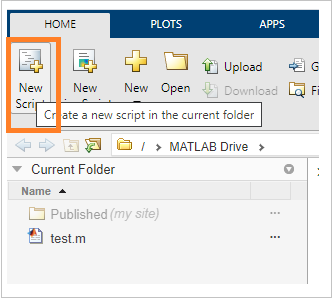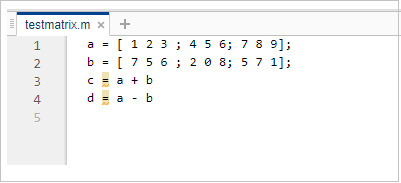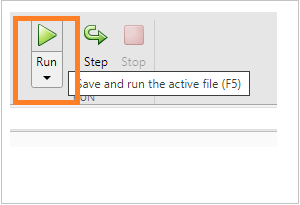# Matlab-Matrix - Working with Matrices

#### Artificial Neural Network and Machine Learning using MATLAB

54 Lectures 4 hours

#### Machine Learning for Data Science using MATLAB

Featured

62 Lectures 9 hours

In this chapter, I will deal with how to run the matrix inside MATLAB Environment to get the output. To define a matrix, and other matrix operations are discussed in details in the next chapters.

To get the matrix output in MATLAB will make use of −

• Command Prompt
• Using m-file

## Using Command Prompt

You can execute matrices directly inside command prompt. Here is an example wherein we have two matrices a and b.

The operation a+b gives the sum of matrix a and b.

The operation a-b gives the subtraction of matrix a and b.

The output is command prompt is as shown below −

```>> a = [ 1 2 3 ; 4 5 6; 7 8 9];
>> b = [ 7 5 6 ; 2 0 8; 5 7 1];
>> c = a + b

c =

8   7   9
6   5  14
12  15  10

>> d = a - b

d =
-6  -3  -3
2   5  -2
2   1   8

>>
```

## Using m-file

You can also make use of a file to write the code and later execute it inside command prompt as shown below −

Click on New script as shown below −This is open a new unsaved file as shown below −Save the file and write your code insideThe file is saved as testmatrix.m.

Now you can use the run button or type the name of the file inside command window.The output will be shown in the command window as shown below −

```>> testmatrix

c =
8   7   9
6   5  14
12  15  10

d =
-6  -3  -3
2   5  -2
2   1   8

>>
```